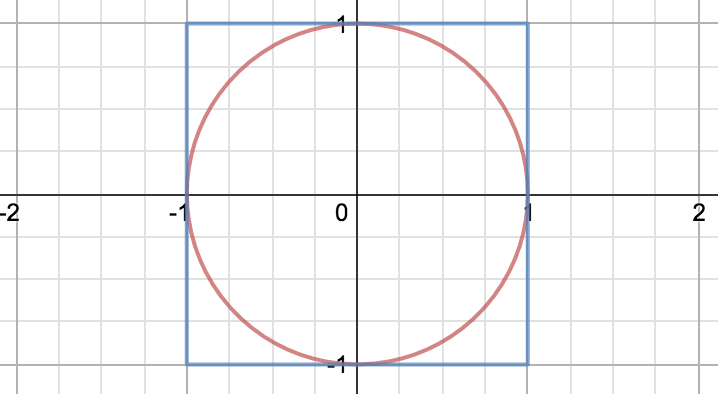the fairmont hairpin, photo by steve harris, cc

The Monte Carlo method describes a class of algorithms which use repeated random samplings to produce statistical or approximate results. A famous algorithm employing such a method, brought to my attention by my friend Jeremy Self recently, is one which calculates the digits of pi.

It starts by considering the ratio between the area of a circle and a bounding rectangle. To make the math easier, you can take the unit circle with radius $$r = 1$$ and a bounding box with sides of length 2, presented below. The area of a circle is $$\pi r^2$$, and a square $$a^2$$, where a is the length of a side. The areas of our unit circle and bounding square are therefore $$\pi$$ and 4, respectively.If we randomly plot points somewhere inside the square, we can expect for the ratio of points inside and outside the circle to be equivalent to the ratio between the areas of the circle and the square, or $$\pi/4$$. The more points we plot, the lower the error rate we should encounter: you can understand this intuitively, as it's possible to plot 5 random points inside the circle and get $$\pi = 4$$, but for 10,000 points it's incredibly unlikely.

We can make the further observation that each quadrant represents an area of a quarter circle and a unit square with the same ratio discussed above, so we can use random samplings where $$0 <= x <= 1$$ and $$0 <= y <=1$$, which works well for lots of random number generators that generate floats in this range.

This is an incredibly simple algorithm to implement, so we decided to have a little shootout and see how the naive solutions fared in multiple languages, running this algorithm with 100,000,000 iterations.

I implemented the algorithm in C, Rust, Go, Python and Ruby, and between Jeremy and the other Jeremy we also had a PHP implementation, which I used below, and a Common Lisp implementation kicking about. The point really wasn't to compare languages to each other; more to challenge our own preconceptions about their performance characteristics.

These are the runtimes on my fairly old Intel I5 750:

CRustGoGo (gccgo) RubyPython2Python3PyPyPHP
2.15 1.70 4.791.96 25.5351.7450.827.2454.6

Despite only testing function call overhead (or compiler inlining), arithmetic, sqrt, and rand, I still learned some interesting things and encountered some surprising results.

There are large differences in the speed of random number generators; having never looked at the implementations of any, I had assumed that most would use the same algorithms. Rust has quite a few at its disposal, and XorShiftRng gave me the fastest results of any language in my tests, while using rng::task_rng() clocked in at 9.45s, slower than PyPy. Since these processes have to seed the generator and produce 200,000,000 random numbers, the tradeoffs made in each RNG implementation can represent huge shifts in elapsed time.

Function calls have surprisingly variable overhead across languages. While I expected that manual inlining of the various in_circle test functions to improve the performance of most languages, in PHP in particular the effect was drastic: runtime drops from 54s to 36s. To compare, the Python runtime only improved by 2s to 48s.

After using Go for so long, I'd blissfully forgotten about compiler optimization flags and other build-time tuning.

When I'd initially implemented the rust version, I used the task_rng random number generator and no optimization switches on rustc, which resulted in a surprisingly high runtime of 24s. Turning on the optimizer lowered that to 9s, nearly 3x improvement. Running with XorShiftRng unoptimized was 9s itself, and flipping on the optimizer got nearly 5x improvement. I'm unsure if this says good things about the optimizer or bad things about the quality of output when not running it.

This made me think to turn on the optimizer for C as well, and finally to try out gccgo and see what it would produce. The runtimes for unoptimized gccgo and C were both around 3.6s, which means that simply adding -O2 to the build roughly doubles performance. Again, this surprised me; I'd always thought, based primarily on ignorance and scoffing at gentoo, that optimizers would produce some incremental improvements at best, and perhaps for general purpose desktop software where you're rarely CPU bound, they do. I wish I had some better knowledge about compiler optimizers to share; for now, these observations will have to suffice.

The star of the show in my mind has to be PyPy: its results on some unmodified, straightforward Python code are pretty astonishing, given the dismal performance of CPython. This is just the sort of thing that should be its bread and butter, but it really came through in a big way. Ruby's results were also quite impressive, as I'd run the Python code first and expected Ruby to be around the same. I was expecting the massive gulf between the scripting and compiled languages, but PyPy shows that this needn't be the case.

These programs represent the tiniest slice of their respective languages, and surely say more about my relative abilities between them than some deeper truths on the language of implementation. Although it should be obvious, I strongly warn against using the results to determine what language to use for a real project, but perhaps this exercise can inspire you to do your own experiments and learn some interesting algorithms in the process!

Oct 19 2014